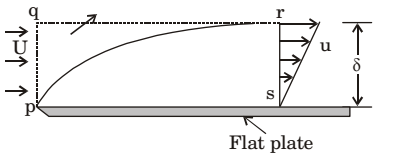#### Fluid Mechanics and Hydraulic Machinery

Direction: A smooth flat plate with a sharp leading edge is placed along a gas stream flowing at U = 10 m/s. The thickness of the boundary layer at section r – s is 10 mm, the breadth of the plate is 1 m (into the paper) and the density of the gas, ρ = 1.0 kg/m3. Assume that the boundary layer is thin, two-dimensional, and follows a linear velocity distribution, u = U (y/δ), at the section r–s, where y is the height from plate.1. The integrated drag force (in N) on the plate, between p – s, is
1. 0.67
2. 0.33
3. 0.17
4. zero
##### Correct Option: C

By momentum equation, we can find drag force.